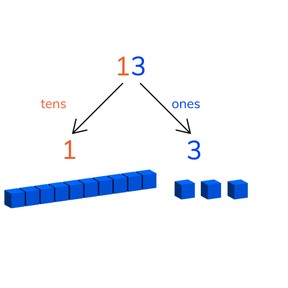Place value- numbers to 20

# Place value- numbers to 20

Place value- numbers to 208,000 schools use Gynzy92,000 teachers use Gynzy1,600,000 students use Gynzy

## General

Students learn place value of numbers to 20. They learn that the numbers 11-20 are made of tens and ones.

K.NBT.A.1

## Relevance

Discuss with students that it is important to know how place value works so they can do calculations later with larger numbers.

## Introduction

The interactive whiteboard has a row of balloons with numbers. Ask students which numbers are missing in the sequence. Repeat this with the row of numbered lamps. The students write down the missing numbers and then tell you which numbers to write in the spaces.

## Development

Using MAB blocks explain what tens and ones are. Show that the numbers 11-20 are made of tens and ones. This is called learning place value of a number. Using the blocks, show what tens are, and ones, and what they make together. Do this to fill in the missing numbers.

Check that students understand how to use place value to build numbers to 20 by asking the following questions:
- What are tens? How do you know?
- What are ones? How do you know?
- Which tens and ones are in the given number?

## Guided Practice

Students first practice combining two numbers with visual support and then do this in the abstract (without support). With the third exercise they fill in the ones, when the tens and total number are given.

## Closing

Check that students understand which tens and ones are needed to create numbers to 20, and how to combine two numbers. Ask students why it is important to be able to do this.

## Teaching Tip

Make sure to emphasize that ten blocks are one tens. The ones are all the loose blocks that come before a tens is made.

## Instruction materials

MAB-blocks

### The online teaching platform for interactive whiteboards and displays in schools

• Save time building lessons

• Manage the classroom more efficiently

• Increase student engagement